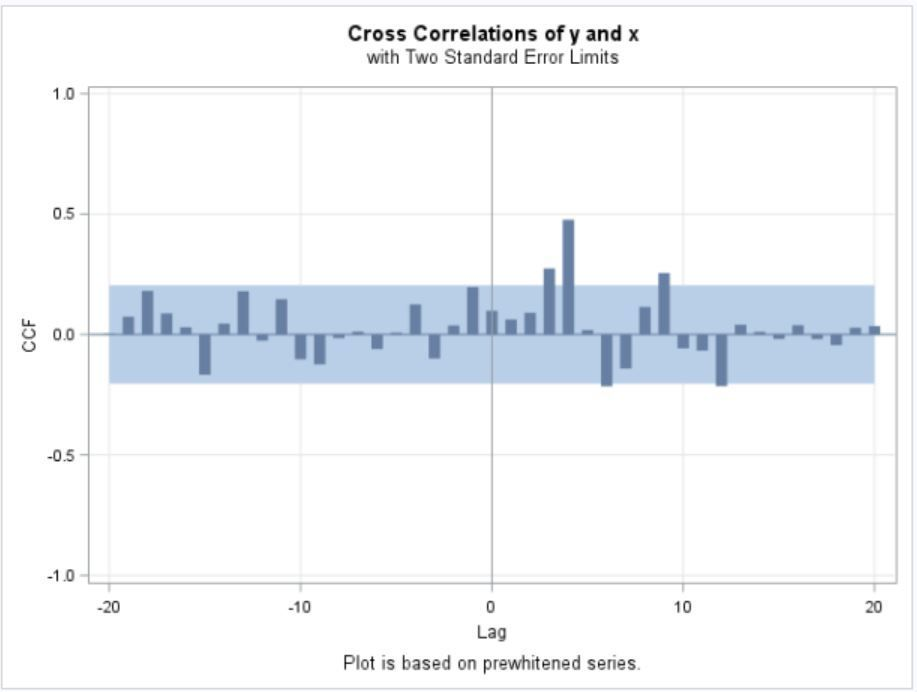## How do I Specify Transfer Functions InputsI am having difficulty coming up with the transfer model inputs. Below is the code that I have so far

```proc arima data = sales plot(unpack);
identify var = x noprint;
estimate q = 2  noprint;
identify var = y crosscorr=(x) nlag = 20;
/*estimate  input = (3\$(2)/(1,1)x) noint printall altparm backlim = -3 plot;*/
/*estimate  input = (3\$(2)/(2)x) noint  printall altparm backlim = -3 plot;*/
estimate  input = (3\$(1)/(1,2)x) noint  printall altparm backlim = -3 plot;
run;
quit;```

My question is how to come up with numerator and denominators based on the graph and differencing. I know b = 3\$ since it is the number of periods it takes before xt affects yt

For s and r , I am confused:

s is from my understanding the number of lags that reside between the first spike and the beginning  of the clear dying down pattern

since I have x and y with no differencing and I set s arbitrarily to s = 2 would I write  input = 3\$(1,2)/(1,2)
r is from my understanding 1 if the lags die down exponentially after the spikes or 2 if they down in a sine wave
since I have x and y with no differencing and since from the graph r = 2 would I input in SAS input = 3\$(1,2)/(1,2)

I am confused on what specifically (1,2) means: does it mean first order differencing and r/s being chose at 2 or does 1,2 mean how many lags before there is a spike

If anyone can help me interpret my graph I would greatly appreciate it .

Thank you

1 ACCEPTED SOLUTION

Accepted Solutions

## Re: How do I Specify Transfer Functions Inputs

Deciding the form of a transfer function relationship is explained in a book by Pankratz, A. (1991). Forecasting with Dynamic Regression Models. New York: John Wiley & Sons.  Also see Pankratz, A. (1983). Forecasting with Univariate Box-Jenkins Models: Concepts and Cases. New York: John Wiley & Sons.  You can also check out professor Hyndman's blog on time series analysis: https://robjhyndman.com/hyndsight/arimax/

Hope this helps.

## Re: How do I Specify Transfer Functions Inputs

Deciding the form of a transfer function relationship is explained in a book by Pankratz, A. (1991). Forecasting with Dynamic Regression Models. New York: John Wiley & Sons.  Also see Pankratz, A. (1983). Forecasting with Univariate Box-Jenkins Models: Concepts and Cases. New York: John Wiley & Sons.  You can also check out professor Hyndman's blog on time series analysis: https://robjhyndman.com/hyndsight/arimax/

Hope this helps.

Discussion stats